## Date and Time in Excel

Dealing with date and time in Excel does not require complicated formula (usually). But it can be tricky and sometimes you just can’t seem to get the right answer in the format you want. We can help you here.

## Formatting Date and Time in Excel

The easiest (and most obvious) way to make sure your cell is in the right format is to use the dropdown box at the top: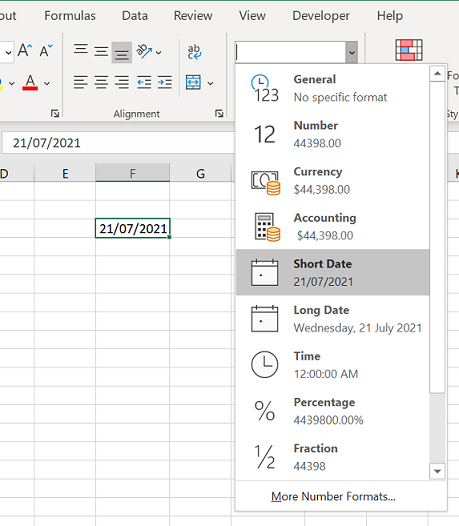If you need more options in terms of formatting, you can select your cell(s) and press Ctrl + 1 (or you can right-click and select Format Cells…)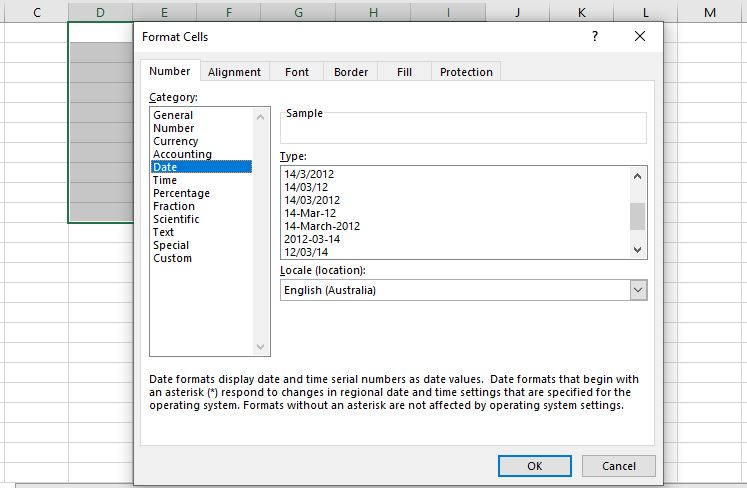For date and time, there is a lot of options under Date, Time and Custom categories.

Lastly if you are very specific with the format you require, the =TEXT(cell, format) formula will be very useful. With this formula, you can have the cell in almost any format you want. E.g. “Wednesday-07-2021”, or “Wednesday 21-July/2021”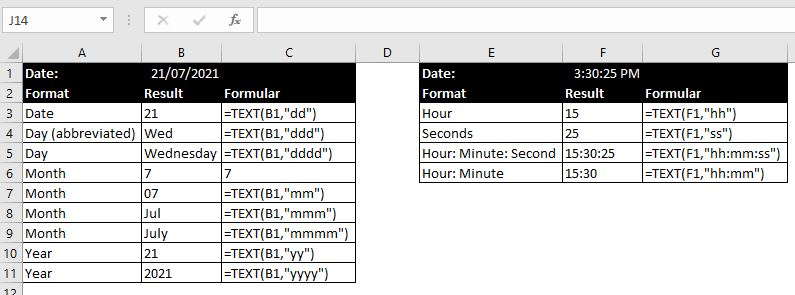And with above, you can be as creative as you want. For example: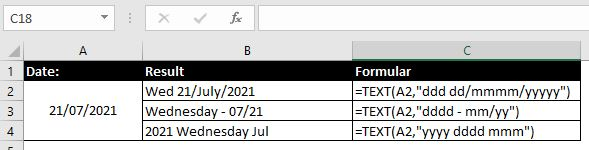## First/Last Working Day of This Month

• Last Working Day of This Month:
• =IF(1<WEEKDAY(EOMONTH(TODAY(),0))<7,EOMONTH(TODAY(),0),WORKDAY(EOMONTH(TODAY(),0),-1))
• There’s a few components in the formula above so let’s break it down
• =TODAY() is a useful formula in Excel that will give you today’s date. And it will of course update automatically everyday when you open the file
• =EOMONTH(TODAY(),0) will return the last day of this month
• =WEEKDAY(date) formula returns a numerical value from 1 to 7 depending on what day of the week the inputted date falls under. 1 = Sunday, 2 = Monday…7 = Saturday
• To summarise the formula above: if the last day of this month falls on Monday to Friday (2 to 6), return the last day of this month. If it falls on Saturday or Sunday (1 or 7), return the previous working day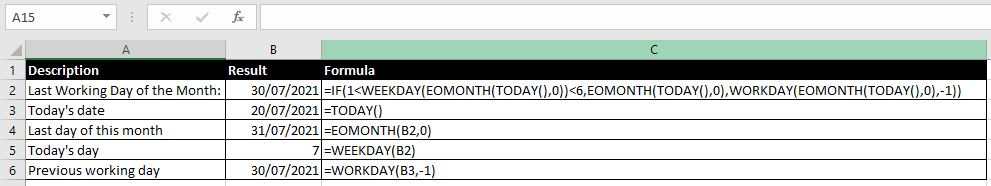## Day/Time Difference

#### Difference In Years/Months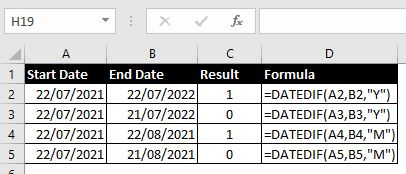=DATEDIF(start date, end date, years/months/days) is a useful formula which allows you to calculate difference between a start date and an end date in years/months/days. If you get a #NUM error, it’s either because:

• Start date is after the end date or
• If we don’t specify whether we want the difference between the two dates in years/months/dates (“y”, “m”, “d”)

#### Difference In Days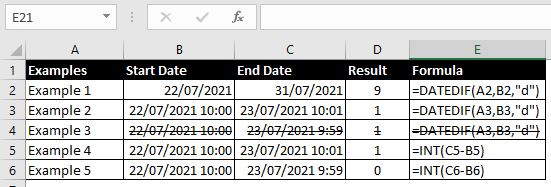It would seem like DATEDIF would be a perfect function to calculate difference between two dates in days. And it is possible as shown above (example 2). But the formula is not perfect when time is involved. Having a look at example 3, 10:00am to 9:59am the next day is not one day but DATEDIF will return 1.

The better way to calculate this would be in examples 4 and 5. What we are doing is simply subtracting the start date from the end date. The INT formula returns the integer part of a number. It does not round up or down. =INT(1.99999) will return 1.

#### Difference In Time

To calculate difference between two times, we can easily do End Time – Start Time. We will just need to understand how to make sense of the result: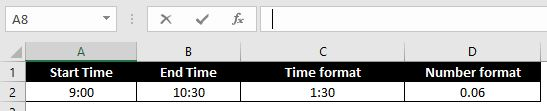Both formulas in C2 and D2 are “=B2 – A2”. By default because A2 and B2 are in time format, the result will also be in a time format (C2). But you can easily change the format to a Number format and in this case this is in cell D2. This format will very often not mean much by itself. But it is easy once we understand that in Excel, 1 as a number represents 1 day. It converts hours to number by putting time as a fraction of 24 hours (a day):

So all we have to do is multiply the result by 24 and we will get the difference in time in a number format in hours. In the example above, (B2 – A2) x 24 = 1.50 which is exactly what we need.

###### ####### Error

There is another advantage in using the number format when calculating difference between two times: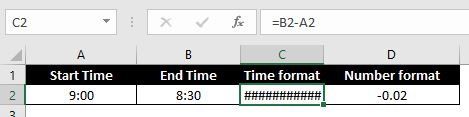When End Time is before the Start Time, we will get a ######## error. But if we convert the result into number format, the error disappears. In the example above, in fact we can put “=(B2 – A2)*24” and we will get -0.50.

##### Various Formats

When we want to add a certain number of hours to a start time to calculate an end time, our first instinct might be to do the following (A2 + B2):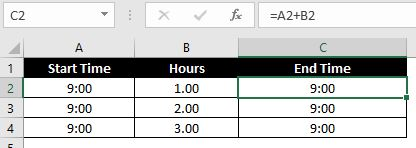As we can see above, it does not work. No matter what number we add to the start time, the end time is always 9:00. This is easy to understand once we remember that Excel converts time to number by putting time as a fraction of 24 hours. So in above, A2 + B2 = 9:00 + One Day which is still 9:00. And of course 9:00 + 2 days (A3 + B3) is still 9:00. And so on with A4 + A5. The correct way would be to first convert hours as a fraction of a day. For example: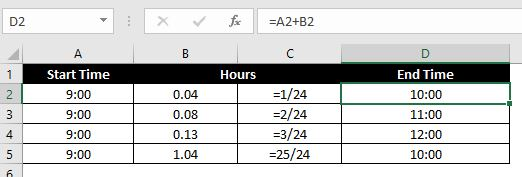And this works just as well if you include dates into start time: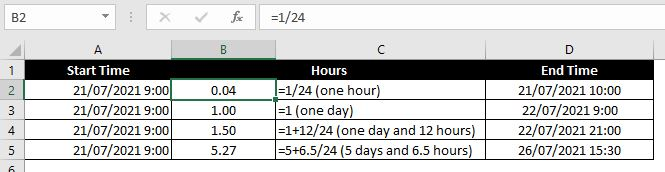## Converting Date to Number

Same as time, we can convert dates to numbers in Excel. 01/01/1900 is the earliest date in Excel. In fact if we enter any date earlier, Excel will automatically put 0/01/1900 which is of course not a valid date: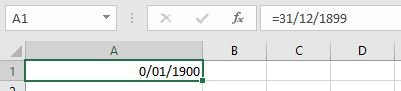So 01/01/1900 is the earliest date in Excel. Excel converts dates to numbers by calculating the number of days from 01/01/1900 to those days with 01/01/1900 being 1, 02/01/1900 being 2, 03/01/1900 being 3 and so on…